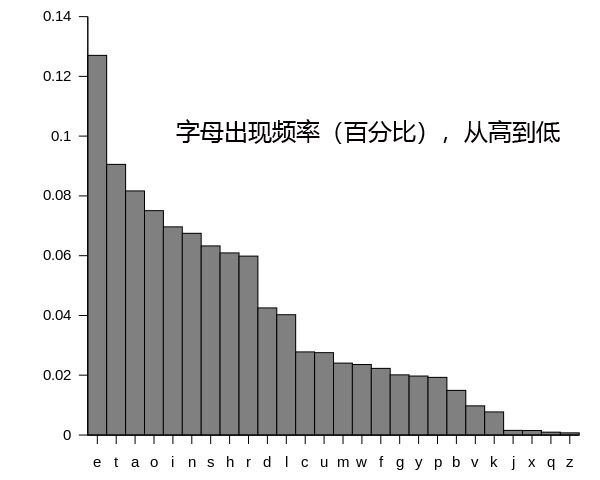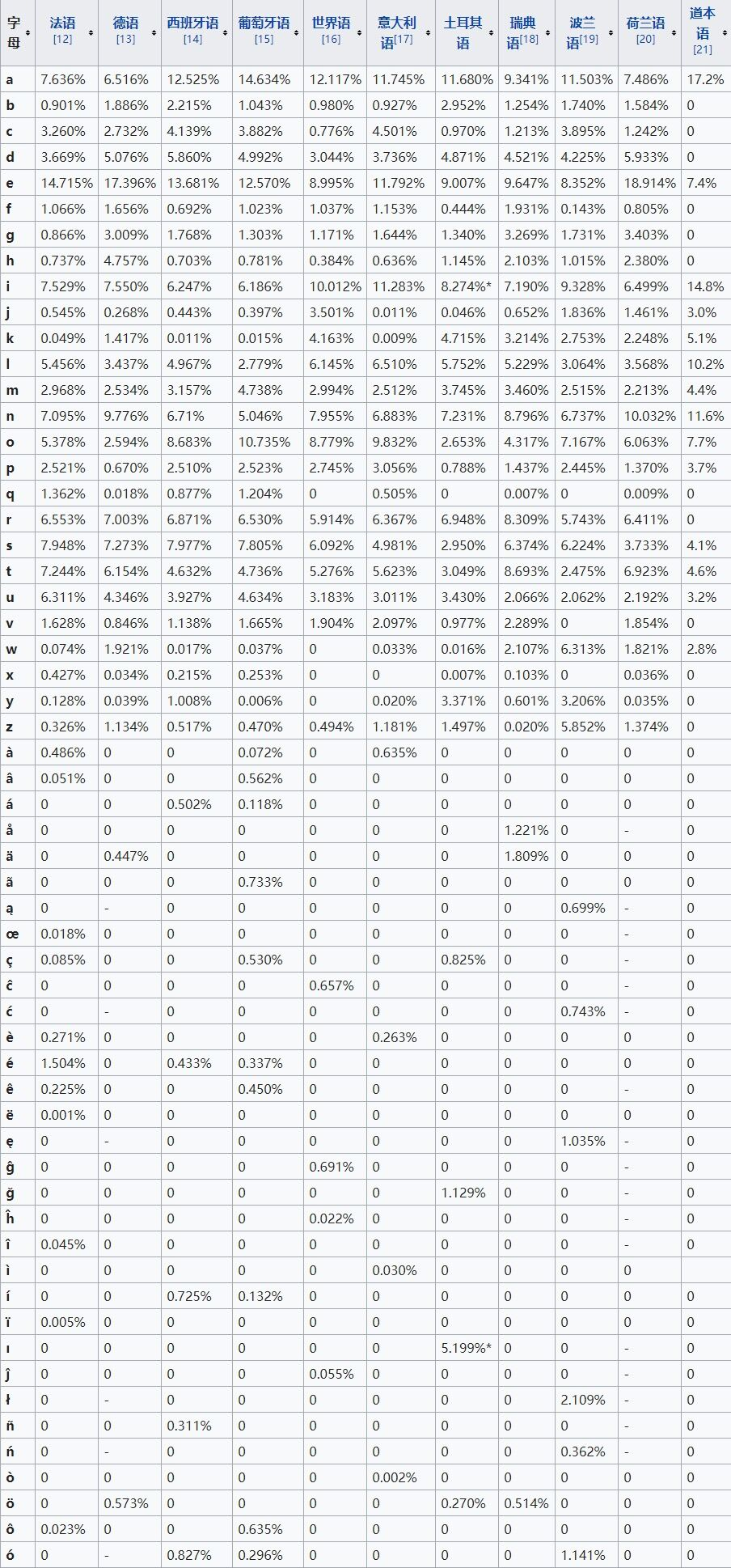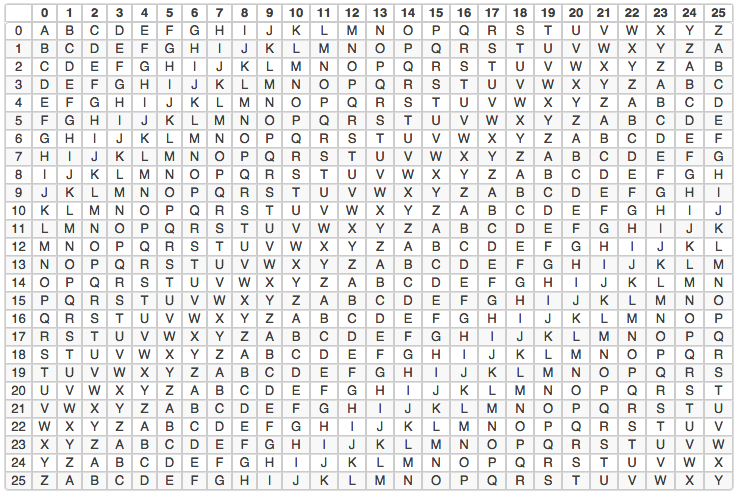# 维吉尼亚密码加解密原理及其实现

 原文 F l y i n t h e s k y 密文 I O B L Q W K H V N B英语中字母频率统计各语言中字母频率统计维吉尼亚密码表

C：密文

P：原文

K：第几套加密方式

 原文 h e l l o w o r l d 密钥 t h i s t h i s t h i 密文 A L T D H E G K S L

C：密文

P：原文

K：第几套加密方式

  1 import java.util.ArrayList;
2 import java.util.Arrays;
3 import java.util.List;
4 import java.util.Optional;
5 /**
6  * 维吉尼亚加密解密
7  * @author 逆熵
8  *
9  */
11
13     private static final String VIRGINIA_PASSWORD_TABLE_ARRAY[] = {"A","B","C","D","E","F","G","H","I","J","K","L","M","N","O","P","Q","R","S","T","U","V","W","X","Y","Z"};
15
16     /**
17      *
18      * 判断对象是否为空，为空返回false，非空返回true
19      * @param object
20      * @return
21      */
22     public static boolean isNotNull(Object object) {
23         return Optional.ofNullable(object).isPresent();
24     }
25
26     /**
27      * 判断字符串非null，并且不为空字符串
28      * @param str
29      * @return
30      */
31     public static boolean isStringNotEmpty(String str) {
32         return isNotNull(str)&&str.trim()!="";
33     }
34
35     /**
36      * C = P + K (mod 26).
37      * 维吉尼亚加密
38      * @param original    原文
39      * @param secretKey    密钥
40      * @return
41      */
42     public static String virginiaEncode(String original,String secretKey) {
43         if(isStringNotEmpty(secretKey)&&isStringNotEmpty(original)){
44             char[] secretCharArray = secretKey.toCharArray();
45             char[] originalCharArray = original.toCharArray();
46             int length = originalCharArray.length;
47
48             List<Integer> list = getSecretKeyList(secretCharArray, length);
49
50             StringBuffer sb = new StringBuffer();
51             for(int m=0;m<length;m++) {
52                 char ch = originalCharArray[m];
54                 if(charIndex==-1) {
55                     sb.append(String.valueOf(ch));
56                     continue;
57                 }
58
60                 //C = P + K (mod 26). 获取偏移量索引
61                 int tmpIndex = (charIndex + list.get(m))%size;
63
64             }
65             return sb.toString();
66         }
67         return null;
68     }
69
70     /**
71      * P = C - K (mod 26).
72      * 维吉尼亚解密
73      * @param cipherText    密文
74      * @param secretKey    密钥
75      * @return
76      */
77     public static String virginiaDecode(String cipherText,String secretKey) {
78         if(isStringNotEmpty(cipherText)&&isStringNotEmpty(secretKey)) {
79             char[] secretCharArray = secretKey.toCharArray();
80             char[] cipherCharArray = cipherText.toCharArray();
81             int length = cipherCharArray.length;
82
83             List<Integer> list = getSecretKeyList(secretCharArray, length);
84             StringBuffer sb = new StringBuffer();
85             for(int m=0;m<length;m++) {
86                 char ch = cipherCharArray[m];
88                 if(charIndex==-1) {
89                     sb.append(String.valueOf(ch));
90                     continue;
91                 }
92
94                 //P = C - K (mod 26). 模逆运算求索引
95                 int len = (charIndex - list.get(m))%size;
96                 //索引小于零，加模得正索引
97                 int tmpIndex = len<0?len+size:len;
99
100             }
101
102             return sb.toString();
103         }
104
105         return null;
106     }
107
108     /**
109      * 获取密钥集合
110      * @param secretCharArray 密钥字符数组
111      * @param length    原文或密文的长度
112      * @return
113      */
114     private static List<Integer> getSecretKeyList(char[] secretCharArray, int length) {
115         List<Integer> list = new ArrayList<Integer>();
116         for (char c : secretCharArray) {
119         }
120
121
122         if(list.size()>length) {
123             //截取和目标原文或密文相同长度的集合
124             list = list.subList(0, length);
125         }else {
126             Integer[] keyArray = list.toArray(new Integer[list.size()]);
127             int keySize = list.size();
128             //整除
129             int count = length/keySize;
130             for(int i=2;i<=count;i++) {
131                 for (Integer integer : keyArray) {
133                 }
134             }
135             //求余
136             int mold = length%keySize;
137             if(mold>0) {
138                 for(int j=0;j<mold;j++) {
140                 }
141
142             }
143         }
144
145         return list;
146     }
147 }

 密钥 K I N G K I N G K I N G K I N G K I N G K I N G K I N G K I N 原文 t h e s u n a n d t h e m a n i n t h e m o o n 密文 D P R C C A K V Q D P R W I A S V Z R M S Y W A

1.从密文中找出拼写完全相同的字符串

2.数第一次到第二次出现中间间隔的字母数

posted @ 2018-12-25 13:34  复姓江山  阅读(20370)  评论(3编辑  收藏